# LOW-DENSITY PARITY-CHECK CODED SIGNALING over a MEMORY CHANNEL

NOTE: This section is Under-Edit if necessary: Construction began on August 2, 2017 and was finished on August 2, 2017.

Low-Density Parity-Check (LDPC) Binary Codes: Signaling over a Rayleigh Fading Memory Channel and Iterative Message-Passing Channel Decoding

```by Darrell A. Nolta
August 2, 2017
```

The AdvDCSMT1DCSS (T1) Professional (T1 Version 2) system tool now offers the capabilities to construct Gallager, Array, and Repeat-Accumulate (RA) Low-Density Parity-Check (LDPC) Binary Codes (Linear Block Codes) and to model and simulate Low-Density Parity-Check (LDPC) Coded Signaling over a Memory Channel (MemC) using these LDPC Codes. In addition to these capabilities, T1 V2 supports the capability of modeling and simulating Multiple Iteration Soft Input/Soft-Decision Output (SISO) LDPC Code Channel Decoding using the Sum-Product Algorithm (SPA). The SPA is a 'symbol-by-symbol' Maximum a Posteriori Probability (MAP) Belief Propagation Algorithm. Also, T1 V2 supports the capability to model and simulate the Multiple Iteration Hard Decision Bit Flipping Algorithm Decoder. These decoding algorithms can be used in the cases for simulated LDPC Coded Signaling over MemC with Additive White Gaussian Noise (AWGN) or LDPC Coded Signaling over Classic Bursty MemC.

This capability of T1 Professional supports decoding of LDPC Coded M-ary Signaling over a Gilbert-Elliot (GE) Burst Channel, Fading Channel (Rayleigh, Rician, and Frequency-Selective), or Intersymbol Interference (ISI) Channel.

T1V2 provides the User the ability to add a Symbol Interleaver-DeInterleaver for the GE Burst Channel or Fading Channel Signaling simulations. In a Fading Channel simulation, a Diversifier-Combiner can be used instead of the Symbol Interleaver-DeInterleaver. In an ISI Channel simulation, a Linear Equalizer can be added.

Also, this capability of T1 Professional supports decoding of LDPC Coded Signaling over a Classic Bursty Channel (CBC) with background error. In a CBC simulation, a Block Interleaver-DeInterleaver can be used.

The SPA Decoder is based on: Model 1 (Bit Messages then Check Messages Iteration Processing) or Model 2 (Check Messages then Bit Messages Iteration Processing); Bit Estimation Likelihood Decision Rule: MAP (Maximum A Posteriori Probability) or ML (Maximum Likelihood) for an Unequiprobable Independent and Identical Distributed (i.i.d.) Binary Source for Rayleigh, Rician, and Frequency-Selective Fading Channel; ML for Equiprobable i.i.d., All-Zero i.i.d., or Markov Binary Source for Burst, Fading, and InterSymbol Interference (ISI) Channel; Implementation Type [for Check Message (Check Node to Bit Node Message) Calculation]: Theoretical SPA, Replace SPA Product Term by Sum, or Replace SPA Product Term by Min-Sum; and User Specified Maximum Number of SPA Decoding Iterations per Block (Imax): 3000 Maximum.

This paper will focus on the application of T1 V2 in the study of the use of Low-Density Parity-Check Coding and Decoding to reduce the Information Bit Errors caused by Rayleigh Fading. Rayleigh Fading is a very important channel impairment that is very destructive in a wireless/mobile communication system that attempts to signal over a dispersive (multipath) environment.

Rayleigh fading channel is a flat (non-selective) fading channel whose parameters vary in time that applies a multiplicative complex Gaussian noise on the transmitted signal. The Rayleigh Fading Channel is a Memory Channel where the physical state of this channel can be characterized by the fading level of a slowly fading transmission path (Robert G. Gallager , page 97). It is important to realize that this channel does not act on each of its inputs in a statistically independent manner. This channel is destructive of the phase information contained within the symbol-to-symbol detection of the channel output signal (i.e., phase variation of the transmitted/received signal). Coherent detection/demodulation cannot be use as long as this phase information cannot be recovered from the received signal.

Given the nature of the Rayleigh Fading Channel, the Frequency Shift Keying (FSK), an orthogonal modulation, is the only modulation to be use for this channel as long as noncoherent demodulation is the only possibility and if the required signal bandwidth is not a limiting factor.

Thus, T1 V2 will offer only FSK modulation when the User selects the Fading Channel for a simulation. And, for the Fading Channel selection, the SPA Decoder uses Energy Detector Demodulation with Fading Statistics.

Consider the SPA Decoding Algorithm Bit Error Rate (BER) or Bit Error Probability Pb performance simulation results for Regular Gallager LDPC Coded (GC) Generator-based Encoding and Signaling that were produced by T1 that are displayed below in Figure 1, 2, 3, 4, 5, 6, and 7 plots for BFSK Modulation. Also, in addition to Pb results, Figure 1, 2, 3, 4, 5, and 6 displays the corresponding Average Number of Iterations per Block (Avg I). For comparison purposes, simulated BER results for UnCoded (UC) and Convolutional Coded (CC) (Rate = 1/2, 64-State) BFSK Signaling are included in each of these figures. Note that this Convolutional code is a NASA standard and provides an excellent reference in regards to its corresponding Viterbi Algorithm (VA) Channel Decoder complexity of 64 trellis states and its resulting BER performance.

Each figure's BER plot displays one or more curves where a curve is constructed from the set of simulated Pb values that correspond to a set of Eb/N0 [Signal-to-Noise Ratio (SNR)] values. Each GC curve displays the performance behavior of a Regular Gallager Coding and SPA Iterative Decoding system example that was used for a T1 V2 simulation.

Figure 1 displays the BER and Avg I versus Eb/N0 for the Maximum Number of Iterations per Block (Imax) of 50 for [Number of Code Word Bits (N) = 20, column weight (j) = 3, row weight (k) = 4] Gallager Coded Signaling for 1,000,006 Equal probable i.i.d. Information (Info) Bits.

Figure 2 displays the BER and Avg I versus Eb/N0 for Imax of 50 for (N = 124, j = 3, k = 4) Gallager Coded Signaling for 1,000,032 Equal probable i.i.d. Info Bits.

Figure 3 displays the BER and Avg I versus Eb/N0 for Imax of 50 for (N = 252, j = 3, k = 4) Gallager Coded Signaling for 1,000,025 Equal probable i.i.d. Info Bits.

Figure 4 displays the BER and Avg I versus Eb/N0 for Imax of 50 for (N = 504, j = 3, k = 4) Gallager Coded Signaling for 1,000,064 Equal probable i.i.d. Info Bits.

Figure 5 displays the BER and Avg I versus Eb/N0 for Imax of 50 for (N = 504, j = 3, k = 6) Gallager Coded Signaling for 1,000,252 Equal probable i.i.d. Info Bits.

Figure 6 displays the BER and Avg I versus Eb/N0 for Imax of 50 for (N = 1008, j = 3, k = 4) Gallager Coded Signaling for 1,000,252 Equal probable i.i.d. Info Bits.

Figure 7 displays the BER versus Eb/N0 for Imax of 50 for (N = 20, j = 3, k = 4; N = 124, j = 3, k = 4; N = 252, j = 3, k =4; N = 504, j = 3, k = 4; N = 504, j = 3, k = 6; N = 1008, j = 3, k = 4) Gallager Coded Signaling for 1,000,006, 1,000,032, 1,000,025, 1,000,064, 1,000,252, and 1,000,252 Equal probable i.i.d. Info Bits, respectively.

One Million plus Info Bits for each Gallager Coded Signaling and SPA Decoding system T1V2 simulation was chosen to allow for reasonable simulation time to generate simulated BER results that can be use to make a 1st order comparison of BER performance between j = 3 Regular Gallager Coded Signaling over a Rayleigh Fading Channel and SPA Decoding. Still, it must be realized that to obtain accurate BER results for long block code lengths, simulation time could take many hours or days (depending on the User's computer). Note that Dr. Gallager discusses this issue in his 1962 paper  and 1963 book .

There are a number of important conclusions that can be drawn from the below displayed simulated Iterative LDPC Code SPA Decoding BER results. It appears that T1 is correctly modeling and simulating LDPC Coded Signaling over a Rayleigh Fading Channel (Memory Channel) with AWGN with SPA Decoding for the selected Regular Gallager Codes.

Each figure of Figure 1 through 6 clearly shows that the reduction in BER as the SNR is increased for the Gallager Coded BFSK Signaling with SPA Decoding with ML Energy Detector Demodulation as compared to the UnCoded BFSK Signaling with Maximum Likelihood (ML) Energy Detector Demodulation. The GC BER curve exhibits the expected behavior as compared to the UC BER curve (i.e. a SNR region where the GC BER is greater than the UC BER, BER is equal to each other, and, then, a SNR region where the GC BER is less than the UC BER). Each figure's GC BER curve exhibits no fluctuations (i.e., a montonically decreasing curve). And one observes that the Average Number of Iterations per Block decrease dramatically and montonically with increasing SNR with no fluctuations.

One can see from Figures 1 through 6 that for SNR greater than 16 dB, the (N = 252, j = 3, k = 4), (N = 504, j = 3, k = 4), (N = 504, j = 3, k = 6), and (N = 1008, j = 3, k = 4) Regular Gallager Codes with SPA Decoding outperforms the CC with VA Decoding with ML Energy Detector Demodulation.

From Figure 7, one can observe that for k = 4 Gallager Codes, the steepness of the BER reduction is increased as the Code Word Length (N) is increased for the region of SNR greater than 16 dB. Also, if we compare BER performance for two different Code Rates (1/4 & 1/2) for N = 504, we see that the Rate ~ 1/4 Gallager Code outperforms the Rate ~ 1/2 Gallager Code.

Based on these BER results displayed in Figures 1 through 7, the BER behavior of SPA Decoding of LDPC Coded Signaling over a Rayleigh Fading Channel (a Memory Channel) with AWGN appears to exhibit the known BER behavior that is observed for SPA Decoding of LDPC Coded Signaling over a coherent Memoryless Channel (MLC) with AWGN (i.e., as the SNR is increased pass a critical value, the BER and the number of iterations starts to dramatically decrease).

But how is this possible?

We must remember that the Sum-Product Algorithm (SPA) decoder makes a decode decision on what most probable code word was transmitted given the channel output signal vector, source, and channel characteristics. This most probable codeword could be selected from (match) one of three possibilities: 1) the transmitted codeword, 2) a non-valid codeword (a 'detected' error codeword), or 3) a valid codeword other than the transmitted codeword (an 'undetected' error codeword).

It attempts to perform this decode decision using an iterating architecture that is based on the MxN Parity-Check Matrix that represents the set of M parity-check equations of a LDPC code. These equations define the BIT NODES, PARITY-CHECK NODES, and these nodes interrelationships (interconnections) architecture. Central to this function of the SPA decoder is that it attempts to solve a set of LDPC code parity-check equations in the process of generating the marginal posterior likelihoods for the code bits.

Key to the successful operation of a SPA Decoder is that its inputs [channel output signal (s) and extrinsic information signals] to BIT NODES are statistically independent (uncorrelated) to each other. This means that the corruption of each signal must not become correlated during the iterative process.

Now, we know that the SPA (a symbol-by-symbol soft-in/soft-out algorithm) uses a procedure to estimate optimally the a posteriori probability (APP) of each Code Bit that is transmitted. It is important to realize that the SPA algorithm is based on the definition that the probability of a particular channel output sequence is a product of individual transition probabilities, i.e., these individual transition probabilities are statistically independent of each other. A MLC satisfies this definition. An AWGN channel is a MLC. The memory channel does not satisfy this definition: the transition probabilities are not statistically independent and, thus, the product of these individual probabilities does not represent the channel output sequence probability.

The Rayleigh Fading Channel without AWGN is a Memory Channel. When AWGN is added to the Rayleigh Fading Channel, the channel output would appear to become statistically independent. This fact may explain why SPA Decoding achieves such good BER results for the Rayleigh Fading Channel with AWGN. We must observe the fact that it is impossible practically to transmit information over a Rayleigh Fading Channel without AWGN. This complex process is a function of the SNR and the 'Rayleigh Fading Channel: Received Information Baseband SNR Loss due to Fading' parameters. But these BER results are not optimal because the SPA Decoder is not an optimal decoder and the Non-Coherent Demodulator does not take into consideration the Markov State structure of the Rayleigh Fading Channel.

Now, the number of Info Bit Errors and the number of 'detected' Errors are indicators of SPA decoder performance for a given simulation SNR. The non-zero BER results are proof that the SPA decoder failed to decode some of the AWGN channel outputs. If the BER is non-zero and no 'Detected' errors have occurred, the SPA decoder had converged to the wrong codeword (s) ('undetected' error events). If we look at the N = 20, j = 3, k = 4 cases, the experimental results inform us that 'undetected' error (s) did occurs at SNR equal to 25 dB. The 'undetected' error (s) did not occur for any other Code Word Length (N = 124, 252, 504, and 1008);

Finally, we summarize that the nature of the SPA Decoder, i.e. its decoding algorithm is suboptimal globally even though the individual decoders (idealized model) are operating optimally. This fact means that there is not a unique solution to the LDPC Decoding problem, i.e., the composite decoder chooses an Estimated Codeword (a block of code bits) out of a set of possible Estimated Codewords. The Stopping Rule halts the decoding for a block when Convergence has occurred (i.e., the product of vest & HT is equal to the NULL Matrix, a bit-by-bit test). Convergence does not imply optimality of an estimated codeword (block) choice and its resulting code bits.

T1 Professional (T1 V2) now offers the LDPC Code (Gallager, Array, and Repeat-Accumulate) construction and LDPC Channel Coding and LDPC Channel Decoding (Iterative; based on the 'Symbol-by-Symbol' MAP Belief Propagation algorithm) for Memory Channel features to the User. The User can choose the simulated Signaling Channel as the Classic Bursty Channel (CBC) with background error or Gilbert-Elliot Burst Channel (GEBC); Rayleigh, Rician, or Frequency-Selective Fading Channel; or PAM or QAM InterSymbol Interference Channel with Linear Equalizer. A Block Interleaver-DeInterleaver (Intl-DeIntl) can be used with CBC or a Symbol Intl-DeIntl can be used with GEBC or Fading Channel. A Diversifier -Combiner can be used for a simulation of Signaling over a Fading Channel.

In conclusion, the User via T1 V2 can get experience with the Generation of LDPC codes and the Sum-Product and Bit Flipping algorithms as applied to Iterative Decoding in simulated digital communication systems for Spacecraft and Mobile Communications and Digital Storage Systems LDPC Coding applications.

References:

 Robert G. Gallager, Information Theory and Reliable Communication, John Wiley & Sons, New York, 1968.

 Robert G. Gallager, "Low-Density Parity-Check Codes," IRE Transactions on Information Theory, Vol. IT-8, pp. 21-28, January 1962.

 Robert G. Gallager, Low-Density Parity-Check Codes, Number 21 of the M.I.T. Press Research Monographs, M.I.T. Press, Cambridge, Massachusetts, 1963.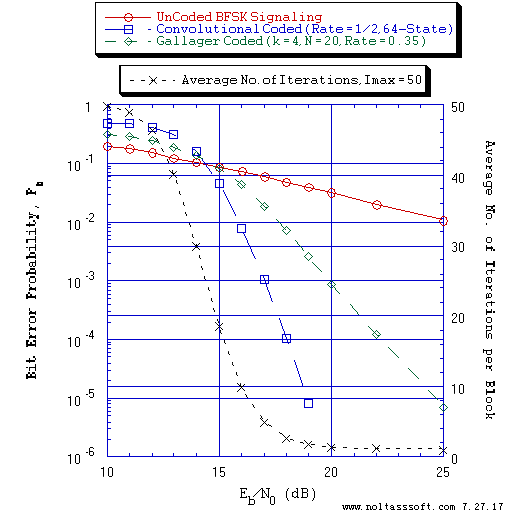```Figure 1. Bit Error Probability for UnCoded, Convolutional, and (N = 20, j = 3,
k = 4) Gallager Coded BFSK Signaling over a Rayleigh Fading Memory Channel
with Additive White Gaussian Noise (AWGN):
```
```          Equal probable Independent and Identical Distributed Source for 10 Million,
1 Million, and 1,000,006 Information Bits for UnCoded, Convolutional Coded,
and Gallager Coded BFSK Signaling respectively over a Vector Channel;
```
```          Rate = 1/2, Constraint Length K = 7 (3,1,0,3,3,2,3) a Best (Optimal)
Non-Recursive Convolutional Code (J.P. Odenwalder) and Viterbi Algorithm
using a Path Memory Length of 35 bits and an Unquantized Branch Metric;
```
```          N = 20, j = 3, k = 4, L = 7, Rate = 0.35 Regular Gallager Code (T1 V2
Computer-generated);
```
```          Rayleigh Fading Channel: Received Information Baseband SNR Loss due to
```
```          Maximum Likelihood (ML) Energy Detector Demodulation; and
```
```          Sum-Product Algorithm Iterative Decoder using Model 2 (Check Messages
then Bit Messages Iteration Processing), Theoretical SPA Implementation
Type, and Maximum Number of Iterations per Block (Imax) = 50.
```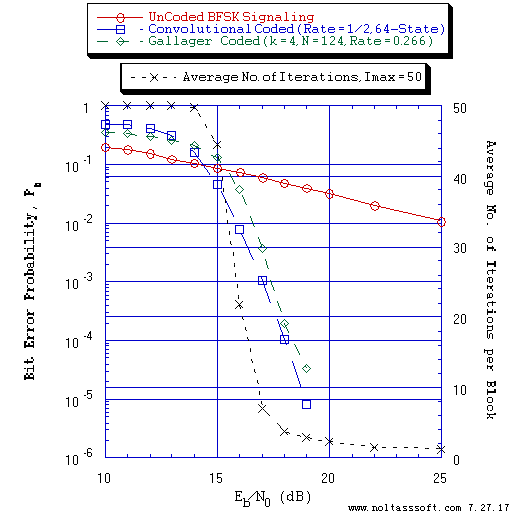```Figure 2. Bit Error Probability for UnCoded, Convolutional, and (N = 124, j = 3,
k = 4) Gallager Coded BFSK Signaling over a Rayleigh Fading Memory Channel
with Additive White Gaussian Noise (AWGN):
```
```          Equal probable Independent and Identical Distributed Source for 10 Million,
1 Million, and 1,000,032 Information Bits for UnCoded, Convolutional Coded,
and Gallager Coded BFSK Signaling respectively over a Vector Channel;
```
```          Rate = 1/2, Constraint Length K = 7 (3,1,0,3,3,2,3) a Best (Optimal)
Non-Recursive Convolutional Code (J.P. Odenwalder) and Viterbi Algorithm
using a Path Memory Length of 35 bits and an Unquantized Branch Metric;
```
```          N = 124, j = 3, k = 4, L = 33, Rate = 0.266 Regular Gallager Code (T1 V2
Computer-generated);
```
```          Rayleigh Fading Channel: Received Information Baseband SNR Loss due to
```
```          Maximum Likelihood (ML) Energy Detector Demodulation; and
```
```          Sum-Product Algorithm Iterative Decoder using Model 2 (Check Messages
then Bit Messages Iteration Processing), Theoretical SPA Implementation
Type, and Maximum Number of Iterations per Block (Imax) = 50.
```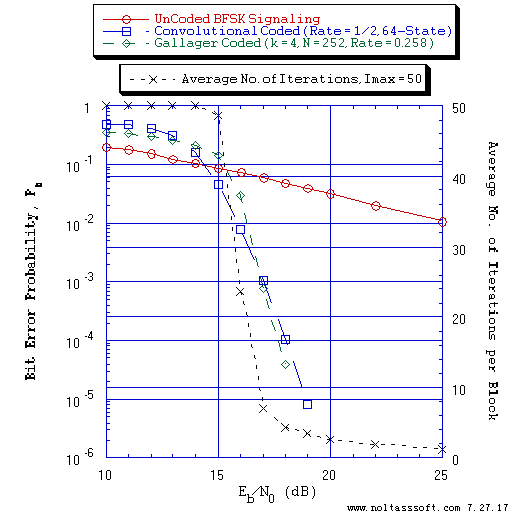```Figure 3. Bit Error Probability for UnCoded, Convolutional, and (N = 252, j = 3,
k = 4) Gallager Coded BFSK Signaling over a Rayleigh Fading Memory
Channel with Additive White Gaussian Noise (AWGN):
```
```          Equal probable Independent and Identical Distributed Source for 10 Million,
1 Million, and 1,000,025 Information Bits for UnCoded, Convolutional Coded,
and Gallager Coded BFSK Signaling respectively over a Vector Channel;
```
```          Rate = 1/2, Constraint Length K = 7 (3,1,0,3,3,2,3) a Best (Optimal)
Non-Recursive Convolutional Code (J.P. Odenwalder) and Viterbi Algorithm
using a Path Memory Length of 35 bits and an Unquantized Branch Metric;
```
```          N = 252, j = 3, k = 4, L = 65, Rate = 0.258 Regular Gallager Code (T1 V2
Computer-generated);
```
```          Rayleigh Fading Channel: Received Information Baseband SNR Loss due to
```
```          Maximum Likelihood (ML) Energy Detector Demodulation; and
```
```          Sum-Product Algorithm Iterative Decoder using Model 2 (Check Messages
then Bit Messages Iteration Processing), Theoretical SPA Implementation
Type, and Maximum Number of Iterations per Block (Imax) = 50.
```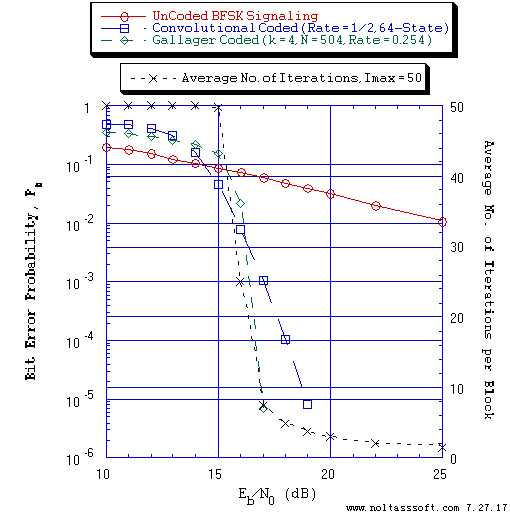```Figure 4. Bit Error Probability for UnCoded, Convolutional, and (N = 504, j = 3,
k = 4) Gallager Coded BFSK Signaling over a Rayleigh Fading Memory
Channel with Additive White Gaussian Noise (AWGN):
```
```          Equal probable Independent and Identical Distributed  Source for 10 Million,
1 Million, and 1,000,064 Information Bits for UnCoded, Convolutional Coded
and Gallager Coded BFSK Signaling respectively over a Vector Channel;
```
```          Rate = 1/2, Constraint Length K = 7 (3,1,0,3,3,2,3) a Best (Optimal)
Non-Recursive Convolutional Code (J.P. Odenwalder) and Viterbi Algorithm
using a Path Memory Length of 35 bits and an Unquantized Branch Metric;
```
```          N = 504, j = 3, k = 4, L = 128, Rate = 0.254 Regular Gallager Code (T1 V2
Computer-generated);
```
```          Rayleigh Fading Channel: Received Information Baseband SNR Loss due to
```
```          Maximum Likelihood (ML) Energy Detector Demodulation; and
```
```          Sum-Product Algorithm Iterative Decoder using Model 2 (Check Messages
then Bit Messages Iteration Processing), Theoretical SPA Implementation
Type, and Maximum Number of Iterations per Block (Imax) = 50.
```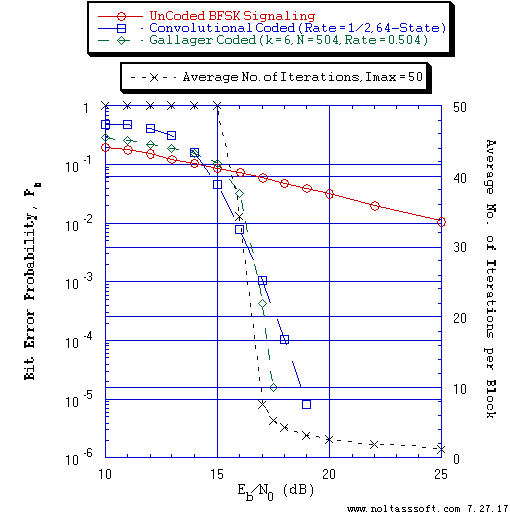```Figure 5. Bit Error Probability for UnCoded, Convolutional, and (N = 504, j = 3,
k = 6) Gallager Coded BFSK Signaling over a Rayleigh Fading Memory
Channel with Additive White Gaussian Noise (AWGN):
```
```          Equal probable Independent and Identical Distributed  Source for 10 Million,
1 Million, and 1,000,252 Information Bits for UnCoded, Convolutional Coded
and Gallager Coded BFSK Signaling respectively over a Vector Channel;
```
```          Rate = 1/2, Constraint Length K = 7 (3,1,0,3,3,2,3) a Best (Optimal)
Non-Recursive Convolutional Code (J.P. Odenwalder) and Viterbi Algorithm
using a Path Memory Length of 35 bits and an Unquantized Branch Metric;
```
```          N = 504, j = 3, k = 6, L = 254, Rate = 0.504 Regular Gallager Code (T1 V2
Computer-generated);
```
```          Rayleigh Fading Channel: Received Information Baseband SNR Loss due to
```
```          Maximum Likelihood (ML) Energy Detector Demodulation; and
```
```          Sum-Product Algorithm Iterative Decoder using Model 2 (Check Messages
then Bit Messages Iteration Processing), Theoretical SPA Implementation
Type, and Maximum Number of Iterations per Block (Imax) = 50.
```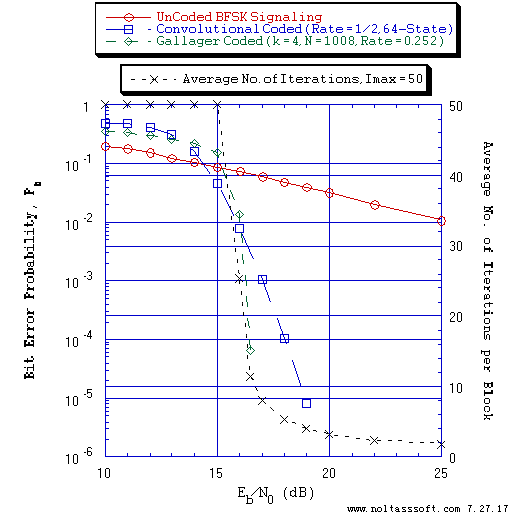```Figure 6. Bit Error Probability for UnCoded, Convolutional, and (N = 1008, j = 3,
k = 4) Gallager Coded BFSK Signaling over a Rayleigh Fading Memory
Channel with Additive White Gaussian Noise (AWGN):
```
```          Equal probable Independent and Identical Distributed  Source for 10 Million,
1 Million, and 1,000,252 Information Bits for UnCoded, Convolutional Coded
and Gallager Coded BFSK Signaling respectively over a Vector Channel;
```
```          Rate = 1/2, Constraint Length K = 7 (3,1,0,3,3,2,3) a Best (Optimal)
Non-Recursive Convolutional Code (J.P. Odenwalder) and Viterbi Algorithm
using a Path Memory Length of 35 bits and an Unquantized Branch Metric;
```
```          N = 1008, j = 3, k = 4, L = 254, Rate = 0.252 Regular Gallager Code (T1 V2
Computer-generated);
```
```          Rayleigh Fading Channel: Received Information Baseband SNR Loss due to
```
```          Maximum Likelihood (ML) Energy Detector Demodulation; and
```
```          Sum-Product Algorithm Iterative Decoder using Model 2 (Check Messages
then Bit Messages Iteration Processing), Theoretical SPA Implementation
Type, and Maximum Number of Iterations per Block (Imax) = 50.
```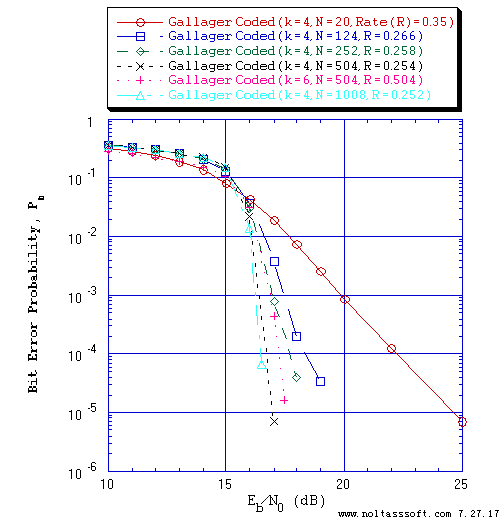```Figure 7. Bit Error Probability for Gallager Low-Density Parity-Check Coded BFSK
Gaussian Noise (AWGN):
```
```          Equal probable i.i.d. Source for 1,000,006, 1,000,032, 1,000,025,
1,000,064, 1,000,252, and 1,000,252 Information (Info) Bits for N = 20;
N = 124; N = 252; N = 504, k = 4; N = 504, k = 6; N = 1008 Regular
Gallager Coded BFSK Signaling respectively over a Vector Channel;
```
```          N = 20, j = 3, k = 4, L = 7, Rate = 0.35 Regular Gallager Code (T1 V2
Computer-generated);
N = 124, j = 3, k = 4, L = 33, Rate = 0.266 Regular Gallager Code (T1 V2
Computer-generated);
N = 252, j = 3, k = 4, L = 65, Rate = 0.258 Regular Gallager Code (T1 V2
Computer-generated);
N = 504, j = 3, k = 4, L = 128, Rate = 0.254 Regular Gallager Code (T1 V2
Computer-generated);
N = 504, j = 3, k = 6, L = 254, Rate = 0.504 Regular Gallager Code (T1 V2
Computer-generated);
N = 1008, j = 3, k = 4, L = 254, Rate = 0.252 Regular Gallager Code (T1 V2
Computer-generated);
```
```          Rayleigh Fading Channel: Received Information Baseband SNR Loss due to
```          Maximum Likelihood (ML) Energy Detector Demodulation; and
```          Sum-Product Algorithm Iterative Decoder using Model 2 (Check Messages